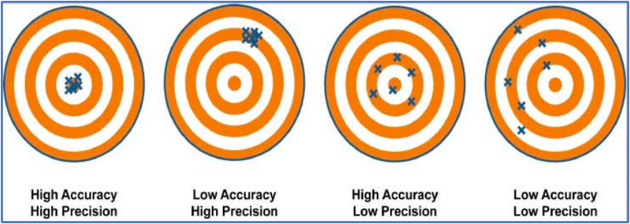# Difference between accuracy and precision## Accuracy

• Accuracy defined as how closely a measured value follows a reference or known value.
• Example: The measurement is said to be inaccurate if you determine in a lab that a substance weighs 3.2 kg when the actual or known weight is 10 kg.
• Accuracy is said as the degree to which measurement results are close to the accepted value.

Accuracy gauges how closely results match the real or predetermined value

## Precision

• The precision is defined as degree to which two or more measurements is matched with each other. A measurement is said to high precise but need not to be accurate.
• Example: If measuring a weight for five times and result is 2.6 kg each time then measurement is very precise. But it cannot be accurate.
• The precision can said to be the extent to which repeated (or reproducible) measurements under the same conditions get the same findings.

## Key difference between Accuracy & Precision

Accuracy is the degree to which the real measurement and the absolute measurement agree whereas precision is the degree of variance between the results of various measurements the same factor.

The closeness of the measurement to the actual measurement is what is meant by accuracy. On the other hand, precision demonstrates how closely a measurement matches those of the other measurements.

Accuracy is the degree of agreement, or how closely a measurement matches an actual measurement. Contrarily, precision is the level of repeatability, which shows why the readings are consistent.

Precision is dependent on multiple factors, whereas accuracy is based on a single factor.

Precision is a measure of statistical unpredictability, whereas accuracy is a measure of statistical bias.

Accuracy concentrated on systematic errors, or those brought on by an instrument’s flaw. As opposed to this precision, random error is an issue. This error occurs frequently without any discernible pattern.

## Bull Eye Representation

The following figure, the bull’s eye represents the accepted true value. In the shown figure, each mark (cross) represents a value repeated in measuring same quantity.

## Example of Accuracy & Precision

Diameter of Coin – A coin has an accepted diameter of 28.054mm. With 2 different observers the measurement are taken. Observer A uses Ruler for measuring while observer B uses micrometer. The readings are as follows.

## Measuring Accuracy & Precision

Observer A – (Plastic Ruler) – Data are 27.9mm, 28.0 mm, 27.8 mm, 28.1 mm

Observer B – (Micrometer) –  Data are 28.246mm, 28.244 mm, 28.246 mm, 28.248 mm

1. Determine  the average value for each set of measurements

Average of Observer A Data =  (27.9 + 28.0 + 27.8 +28.1)/4

= 27.95 = 28.0 mm

Average of Observer B Data = (28.246 + 28.244 + 28.246 + 28.248)/4

= 28.246 mm

1. Determine the % error for each set of measurements.

% Error from observer A = {(28.0 – 28.05)/28.05} X 100  = 0.18%

% Error from observer A = {(28.246 – 28.05) / 28.05} X 100  = 0.86%

1. Evaluate each set’s average value against the accepted value and conclude which observer data is more accurate & more precise.

From calculation the average value of 28.0 mm is closer to the accepted value of 28.05 mm. Therefore Observer A is more accurate than observer B.

From measured data observer B values are closer to the average value, therefore observer B is more precise.

1. Analyze the percentage error for each set of values
• From the calculation of error, observer A has an error of 0.18% is smaller than observer B’s value of 0.86%, therefore observer A is said to be more accurate.
• The precision parameter cannot be determined based on error percentage. But range of values are essential for determining precision.

## What Causes Accuracy?

• Within the instrument’s operational range, erroneous values generate significant errors
• The effectiveness of calibrators may have a direct impact on the outcomes of instrument calibration. For consistent findings and performance, only calibrators designed to express tolerances and standards should be used.
• The past errors in the instrument and internal control procedures are another tolerance connected to the formulation and design a calibrator.
• Ambient conditions including pressure, temperature and humidity have an impact on accuracy. Instruments should be calibrated in a settling similar to the one in which they will be used.

## What causes precision?

Regardless of precision and accuracy, all measurements are subject to some degree of uncertainty

This is due to 2 factors.

• Measuring instrument limitation (systematic error)
• Measurement skills of the experimenter (random error)

## When we can get high accuracy and high precision?

When experimental results is equal to theoretical results then measurement has high accuracy.

When experiment is precise as the numerous trials yields similar results with small variation then experiment is said to be high precision .

## What factors affect accuracy & precision in measurement?

• The quality of the device.

• The reaction of the metals used in the device with the test solutions or samples.

• Faults that occur in the machinery or the apparatus.

## List some methods to improve accuracy & precision

• Perform routine maintenance
• Proper interval calibration
• Consider human factor
• Analysis system measurement by taking repeated measurements & understand essential data.